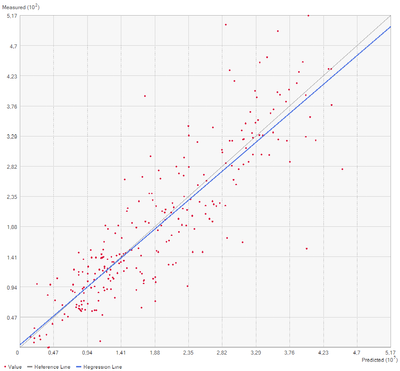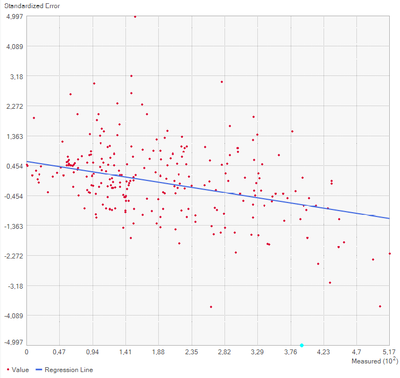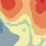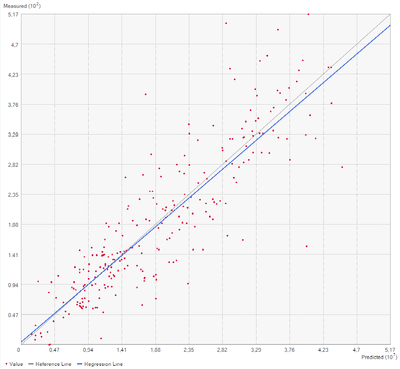# Contradicion in cross validation plots

384
6
06-02-2021 09:42 AM
Labels (1)New Contributor II

The cross validation plot shows that high measurements (~between 3 and 5) are overestimated (blue line below grey, predicted on x-axis, measured on y-axis):The standardized error plot indicates underestimation for the same data and value range (~between 3 and 5):I may misinterpret these plots, but this seems contradictory.

The cross validation was carried out in ArcGIS Pro 2.5.0.

Thanks!

Christian

Tags (2)
6 RepliesbyMVP Esteemed Contributor

Is this related to methods in the Geostatistical Analyst?

Cross Validation (Geostatistical Analyst)—ArcGIS Pro | Documentation

... sort of retired...New Contributor II

Geostatistical Wizard > Kriging/CoKriging > Ordinary Kriging (Prediction)

I guess this is part of the Geostatistical Analyst Extension?byEsri Regular Contributor

Hi Christian,

I think the slopes of the blue trend lines is causing the confusion.  If you ignore the blue lines for a minute, notice in the first graph that most of the largest measured values (the points highest on the y-axis) generally fall above the 1:1 gray line, indicating that they are underpredicted (ie, the prediction is less than the measured value).  The corresponding points on the Standardized Error graph (the points furthest on the right) generally do have negative standardized errors, indicating that they were underpredicted. Ignoring the trend lines, I hope you can see that the scatterplots and their values do not contradict each other.

The blue trend lines, however, add some confusion.  It does seem contradictory that the blue line in the first graph would be flatter than the 1:1 gray line while the trend line of the second graph has a negative slope.  The latter seems to indicate smoothing, and the former seems to indicate the opposite.

There are a couple things that come to mind for how this could happen. First, standardized errors are scaled by the inverse of the standard deviation, so the relative impact on the trend line for each point is different between each graph, which could be enough to change the slope of the Standardized Error trend line from positive to negative.  Please look to Error graph, which shows the equivalent scatterplot and trend line for the unstandardized errors, and see if its trend line is positive or negative.

Second, the blue trend lines use a robust estimation technique and are not just simple linear regressions.  The documentation describes this process as: "This procedure first fits a standard linear regression line to the scatterplot. Next, any points that are more than two standard deviations above or below the regression line are removed, and a new regression equation is calculated."  This removal of values could be subtle enough to switch the sign of the slope.

-EricNew Contributor II

Hi Eric,
thanks for your detailed explanations! I agree that the blue line is the problem. It seems like the regression does not properly represent the point cloud. Your explanation (outliers > 2 standard deviations ignored) makes sense though. However, this makes results quite counterintuitive. Particular because the same procedure appears to have not the same effect in the standard error plot causing some ambiguity.
Moreover, in older version of ArcGIS the cross validation plot showed ‘predicted’ on the y-axis and ‘measured’ on the x-axis. This is why I expected some kind of bug.
Regards,
ChristianbyEsri Regular Contributor

Hi again,

You're correct that in ArcMap, the Measured value appears on the x-axis, but this was changed for ArcGIS Pro, based on new research in 2008.  You can read the full details of why the Measured value should be on the y-axis in this paper.  Long story short, the axes don't matter for the R^2 value and for testing whether the slope is equal to 0.  However, to test that the line has slope=1 and y-intercept=0, the predicted values need to be on the x-axis and measured values on the y-axis.  This is only relevant for Measured vs Predicted, so we kept the Measured value on the x-axis for all other graphs.

I'm still interested to hear whether the Error plot also has a negative slope.  These are the raw errors that are not standardized by the kriging variance, so that graph may still have a positive slope even if the standardized errors have a negative slope.

-EricNew Contributor II

The unstandardized error plot has also a negative slope:Which in turn contradicts a slope < 1 in the unstandardized predicted-measured plot:Some outliers may have been ignored in the regression (sigma > 2). But why only in this plot?

Christian